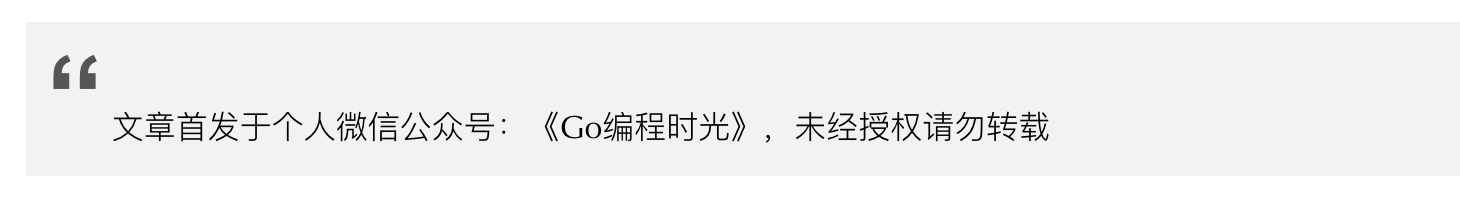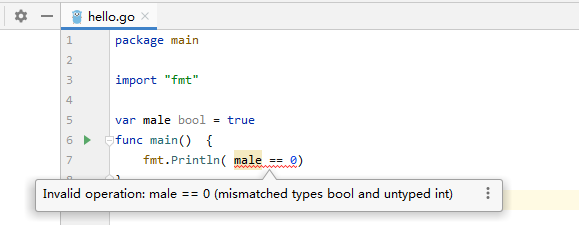# 1.6 数据类型：字典与布尔类型¶## 1. 字典¶

```map[KEY_TYPE]VALUE_TYPE
```

### 声明初始化字典¶

```// 第一种方法
var scores map[string]int = map[string]int{"english": 80, "chinese": 85}

// 第二种方法
scores := map[string]int{"english": 80, "chinese": 85}

// 第三种方法
scores := make(map[string]int)
scores["english"] = 80
scores["chinese"] = 85
```

```import "fmt"

func main() {
// 声明一个名为 score 的字典
var scores map[string]int

// 未初始化的 score 的零值为nil，无法直接进行赋值
if scores == nil {
// 需要使用 make 函数先对其初始化
scores = make(map[string]int)
}

// 经过初始化后，就可以直接赋值
scores["chinese"] = 90
fmt.Println(scores)
}
```

### 字典的相关操作¶

```scores["math"] = 95
```

```scores["math"] = 100
```

```fmt.Println(scores["math"])
```

```delete(scores, "math")
```

```package main

import "fmt"

func main() {
scores := make(map[string]int)
fmt.Println(scores["english"]) // 输出 0
}
```

### 判断 key 是否存在¶

```import "fmt"

func main() {
scores := map[string]int{"english": 80, "chinese": 85}
math, ok := scores["math"]
if ok {
fmt.Printf("math 的值是: %d", math)
} else {
fmt.Println("math 不存在")
}
}
```

```import "fmt"

func main() {
scores := map[string]int{"english": 80, "chinese": 85}
if math, ok := scores["math"]; ok {
fmt.Printf("math 的值是: %d", math)
} else {
fmt.Println("math 不存在")
}
}
```

### 如何对字典进行循环¶

Go 语言中没有提供类似 Python 的 keys() 和 values() 这样方便的函数，想要获取，你得自己循环。

1. 获取 key 和 value

```import "fmt"

func main() {
scores := map[string]int{"english": 80, "chinese": 85}

for subject, score := range scores {
fmt.Printf("key: %s, value: %d\n", subject, score)
}
}
```
1. 只获取key，这里注意不用占用符。

```import "fmt"

func main() {
scores := map[string]int{"english": 80, "chinese": 85}

for subject := range scores {
fmt.Printf("key: %s\n", subject)
}
}
```
1. 只获取 value，用一个占位符替代。

```import "fmt"

func main() {
scores := map[string]int{"english": 80, "chinese": 85}

for _, score := range scores {
fmt.Printf("value: %d\n", score)
}
}
```

## 2. 布尔类型¶

```>>> True == 1
True
>>> False == 0
True
>>>
```Go 中确实不如 Python 那样灵活，bool 与 int 不能直接转换，如果要转换，需要你自己实现函数。

bool 转 int

```func bool2int(b bool) int {
if b {
return 1
}
return 0
}
```

int 转 bool

```func int2bool(i int) bool {
return i != 0
}
```

```import "fmt"

var male bool = true
func main()  {
fmt.Println( !male == false)
// 或者
fmt.Println( male != false)
}

// output: true
```

```>>> age = 15
>>> gender = "male"
>>>
>>> gender == "male" and age >18
False
```

```import "fmt"

var age int = 15
var gender string = "male"
func main()  {
//  && 两边的表达式都会执行
fmt.Println( age > 18 && gender == "male")
// gender == "male" 并不会执行
fmt.Println( age < 18 || gender == "male")
}

// output: false
// output: true
```